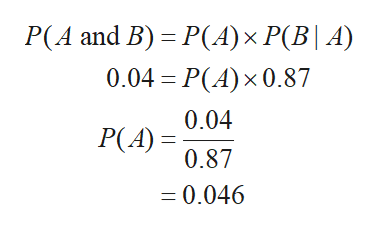# Given P (A and B) 00.04, P (B) 0.64, P (B|A) 0.87, what is P (A)?

Question
6 viewshelp_outlineImage TranscriptioncloseGiven P (A and B) 0 0.04, P (B) 0.64, P (B|A) 0.87, what is P (A)? fullscreen
check_circle

Step 1

Conditional probability:

The conditional probability describes that the probability of an event B occurs given another event A has already occurred. Usually, the conditional probability is denoted as P(B|A).

Step 2

Computation of P(A):

Suppose, the two events A and B are not independent, then the probability of A and B is defined as follows.

P(A and B) = P(A) × P(B|A)

Here, P(A and B) is 0.04 and P(B|A) is 0.87.

Substituting the values in the above formu...help_outlineImage TranscriptioncloseР(А and B) - P(А)х Р(B| A) 0.04 - Р(A) x0.87 0.04 Р(A) 3D 0.87 =0.046 fullscreen

### Want to see the full answer?

See Solution

#### Want to see this answer and more?

Solutions are written by subject experts who are available 24/7. Questions are typically answered within 1 hour.*

See Solution
*Response times may vary by subject and question.
Tagged in

### Other